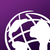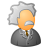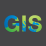# How to Query a Feature Layer with an Intersecting Point?

3589
5
04-02-2018 08:41 AMNew Contributor II

I have a point, created with arcgis.geometry.Point, that I wish to use in a feature layer query to only return the records that intersect that point.  I can do this via the REST API by setting the geometry parameters, but I am struggling to figure out how to do this with the Python API.  I have read the reference guide and examples, but still not sure how to do this.

Any help would be much appreciated.

1 Solution

Accepted SolutionsOccasional Contributor

Sorry for confusion with arcpy instead Python API

As per Python API, we can use the parameter for geometry_filter

`query`(layer_defs_filter=None, geometry_filter=None, time_filter=None, return_geometry=True, return_ids_only=False, return_count_only=False, return_z=False, return_m=False, out_sr=None)

define geometry filter to use as parameter for feature_layer.query method

arcgis.geometry.filters.contains(point)

5 RepliesOccasional Contributor

To do same in Python, create the PointGeometry first

point = arcpy.Point(x, y)

pointGeometry = arcpy.PointGeometry(point)

Apply the same PointGeometry in SelectLayerByLocation_management

arcpy.SelectLayerByLocation_management('query_feature_layer', 'CONTAINS', pointGeometry)New Contributor II

Thank you for the response.

I am trying to do this through the Python API, not with arcpy.  Apologies if I did not make this clear in my question.  I am wondering if there is a way to query the feature layer and include the point to only return back records that intersect.  Something like:

`query_result = feature_layer.query(where='1=1', some geometry parameter)`

Using the REST API, I would construct a query like this to only get back the record that intersects the point: https://services6.arcgis.com/bxsVBCAOuftUsVxD/arcgis/rest/services/California_Cities/FeatureServer/0...Occasional Contributor

Sorry for confusion with arcpy instead Python API

As per Python API, we can use the parameter for geometry_filter

`query`(layer_defs_filter=None, geometry_filter=None, time_filter=None, return_geometry=True, return_ids_only=False, return_count_only=False, return_z=False, return_m=False, out_sr=None)

define geometry filter to use as parameter for feature_layer.query method

arcgis.geometry.filters.contains(point)byEsri Esteemed Contributor

Hi Mike,

Here's an example:

``````from arcgis.gis import GIS
from arcgis.geometry import filters
gis = GIS('https://www.arcgis.com', 'agol', 'gis123')

ptFeatureLayer = gis.content.get('11ed3710e5a54d988d9e4f9d4068ca6f')

ptFeatures = ptFeatureLayer.layers
queryResult = ptFeatures.query(where='OBJECTID=10')
ptFeat = queryResult.features.geometry

polygonFeatures = polygonFeatureLayer.layers
queryResult = polygonFeatures.query(where='1=1', return_ids_only=True, geometry_filter=filters.intersects(ptFeat))
print(queryResult)‍‍‍‍‍‍‍‍‍‍‍‍‍‍``````New Contributor II

Thank you, this worked great.  I used intersect to get the results I needed.

point = arcgis.geometry.Point({"x" : x, "y" : y, "spatialReference" : {"wkid" : 4326}})
gf = arcgis.geometry.filters.intersects(point)
test = cities_layer.query(where='1=1',geometry_filter=gf)